Поиск даташита (1.687.043 компонентов) Где искатьв наименованиив описанииDatasheet: 2SC4813 (NEC)

Npn Silicon Epitaxial Transistor For High-speed Switching
Скачать:PDFZIPDocument Outline

• COVER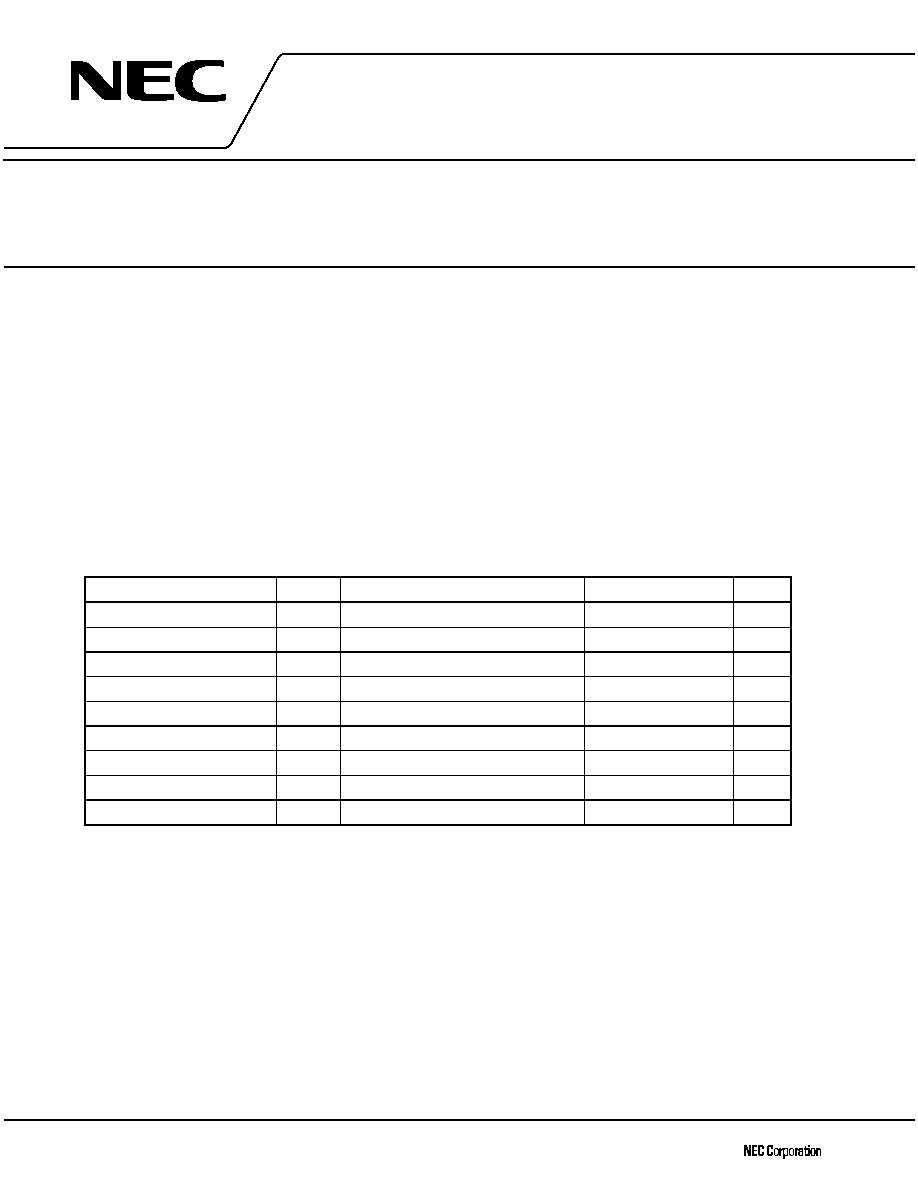The information in this document is subject to change without notice. Before using this document, please
Not all devices/types available in every country. Please check with local NEC representative for
1998
Document No. D15603EJ2V0DS00 (2nd edition)
Date Published April 2002 N CP(K)
Printed in Japan
SILICON POWER TRANSISTOR
2SC4813
NPN SILICON EPITAXIAL TRANSISTOR
FOR HIGH-SPEED SWITCHING
DATA SHEET
2002
The 2SC4813 is a power transistor developed for high-speed switching and features high h
FE
and low V
CE(sat)
.
This transistor is ideal for use as a driver in DC/DC converters and actuators.
In addition, this transistor features a package that can be auto-mounted in radial taping specifications, thus
contributing to mounting cost reduction.
FEATURES
� Low V
CE(sat)
: V
CE(sat)
0.3 V
@I
C
= 3.0 A, I
B
= 30 mA
� High h
FE
: h
FE
= 450 to 2,000 @V
CE
= 2.0 V, I
C
= 3.0 A
� On-chip dumper-diode
� Auto-mounting possible in radial taping specifications
ABSOLUTE MAXIMUM RATINGS (Ta = 25

C)
Parameter
Symbol
Conditions
Ratings
Unit
Collector to base voltage
V
CBO
100
V
Collector to emitter voltage
V
CEO
100
V
Emitter to base voltage
V
EBO
7.0
V
Collector current (DC)
I
C(DC)
�7.5
A
Collector current (pulse)
I
C(pulse)
PW
10 ms, duty cycle 2%
�10
A
Base current (DC)
I
B(DC)
2.0
A
Total power dissipation
P
T
Ta = 25
�C
1.8
W
Junction temperature
T
j
150
�C
Storage temperature
T
stg
-55 to +150
�C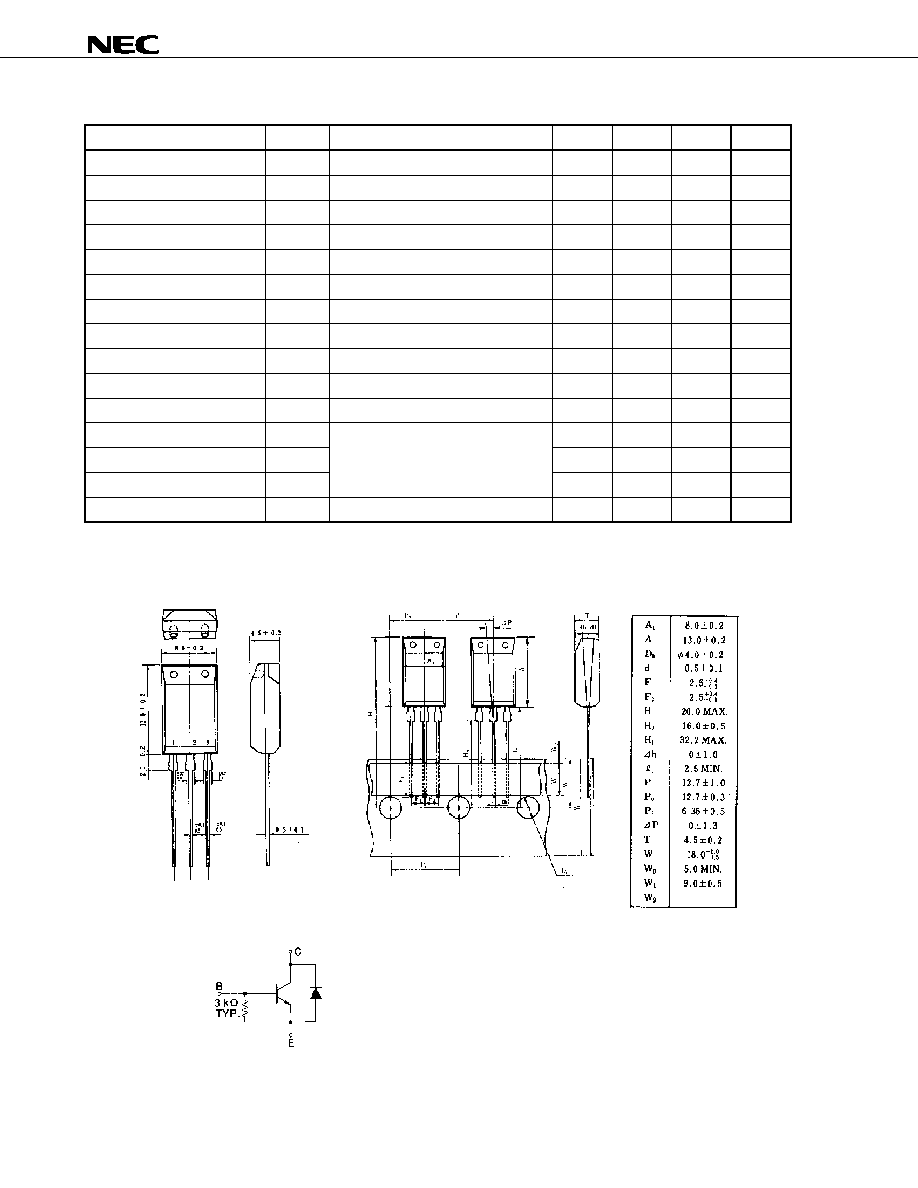Data Sheet D15603EJ2V0DS
2
2SC4813
ELECTRICAL CHARACTERISTICS (Ta = 25

C)
Parameter
Symbol
Conditions
MIN.
TYP.
MAX.
Unit
Collector cutoff current
I
CBO
V
CB
= 100 V, I
E
= 0
10
�A
Emitter cutoff current
I
EBO
V
EB
= 5.0 V, I
C
= 0
17
mA
DC current gain
h
FE1
*
V
CE
= 2.0 V, I
C
= 3.0 A
450
2,000
-
DC current gain
h
FE2
*
V
CE
= 2.0 V, I
C
= 5.0 A
150
-
Collector saturation voltage
V
CE(sat)1
*
I
C
= 3.0 A, I
B
= 60 mA
0.1
0.2
V
Collector saturation voltage
V
CE(sat)2
*
I
C
= 3.0 A, I
B
= 30 mA
0.15
0.3
V
Collector saturation voltage
V
CE(sat)3
*
I
C
= 5.0 A, I
B
= 100 mA
0.4
V
Collector saturation voltage
V
CE(sat)4
*
I
C
= 5.0 A, I
B
= 50 mA
0.55
V
Base saturation voltage
V
BE(sat)
*
I
C
= 5.0 A, I
B
= 50 mA
1.2
V
Gain bandwidth product
f
T
V
CE
= 5.0 V, I
C
= 1.0 A
150
MHz
Collector capacitance
C
ob
V
CB
= 10 V, I
E
= 0 , f = 1 MHz
110
pF
Turn-on time
t
on
0.5
�s
Storage time
t
stg
2.0
�s
Fall time
t
f
I
C
= 5.0 A, I
B1
=
-I
B2
= 100 mA
R
L
= 3.0
, V
CC
16 V
0.5
�s
Diode order voltage
V
DF
I
DF
= 5.0 A
1.4
V
* Pulse test PW
350
�s, duty cycle 2%
PACKAGE DRAWING (UNIT: mm)
TAPING SPECIFICATION
Electrode Connection
1. Base 2. Collector 3. Emitter
EQUIVALENT CIRCUIT
0.7 MAX.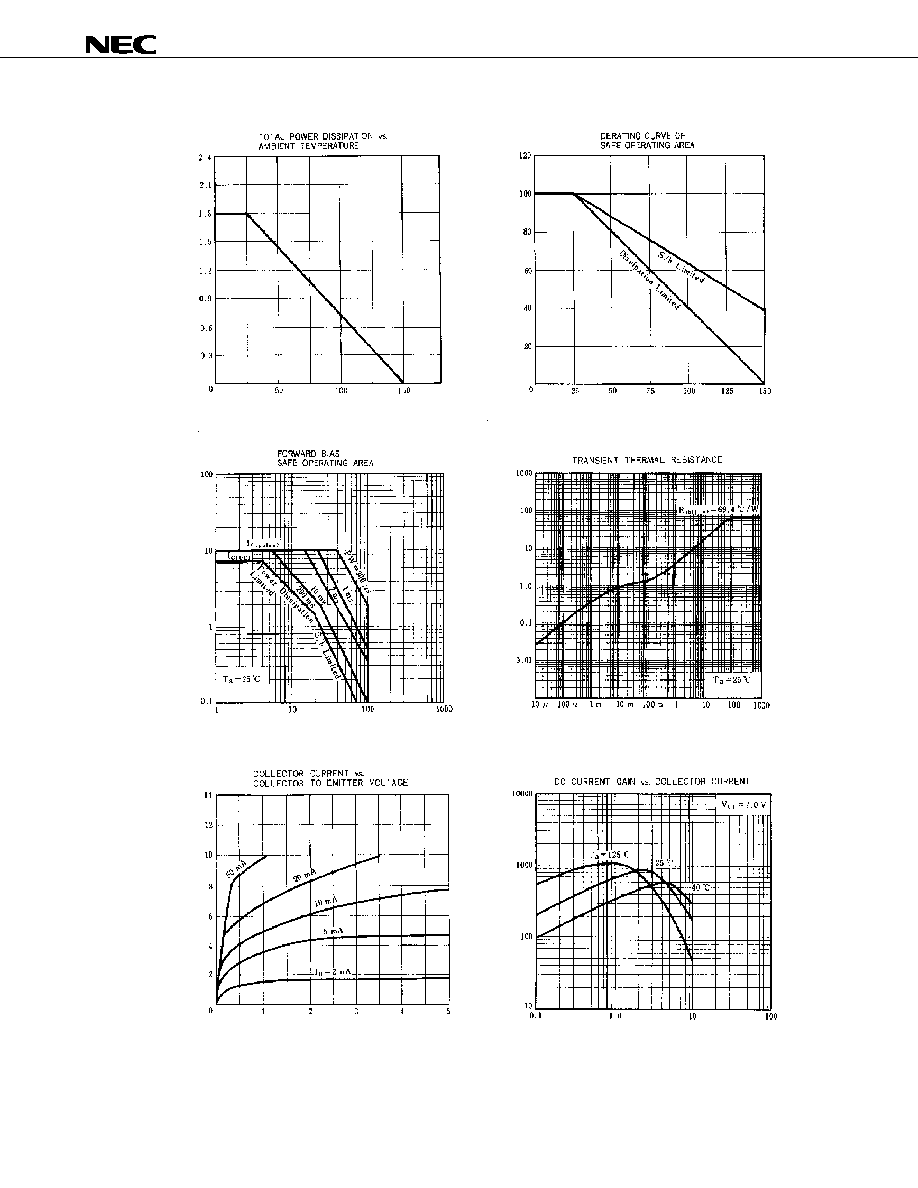Data Sheet D15603EJ2V0DS
3
2SC4813
TYPICAL CHARACTERISTICS (Ta = 25

C)
T
o
t
a
l

P
o
w
e
r D
i
s
s
ip
at
io
n

P
T
(W
)
Ambient Temperature Ta (
�C)
Collector to Emitter Voltage V
CE
(V)
Ambient Temperature Ta (
�C)
I
C
D
e
r
a
ti
n
g
d
T
(
%
)
Col
l
e
c
t
or
Cur
r
e
n
t I
C
(A
)
Pulse Width PW (s)
Collector to Emitter Voltage V
CE
(V)
Collector Current I
C
(A)
Single pulse
T
r
ans
ient T
h
e
r
m
a
l Res
i
s
t
an
c
e

r
th
(j
-
a
)
(t
)
(

C/
W
)
DC Cu
r
r
e
n
t Ga
i
n

h
FE
Col
l
e
c
t
or

Cur
r
e
n
t I
C
(A
)
Single pulse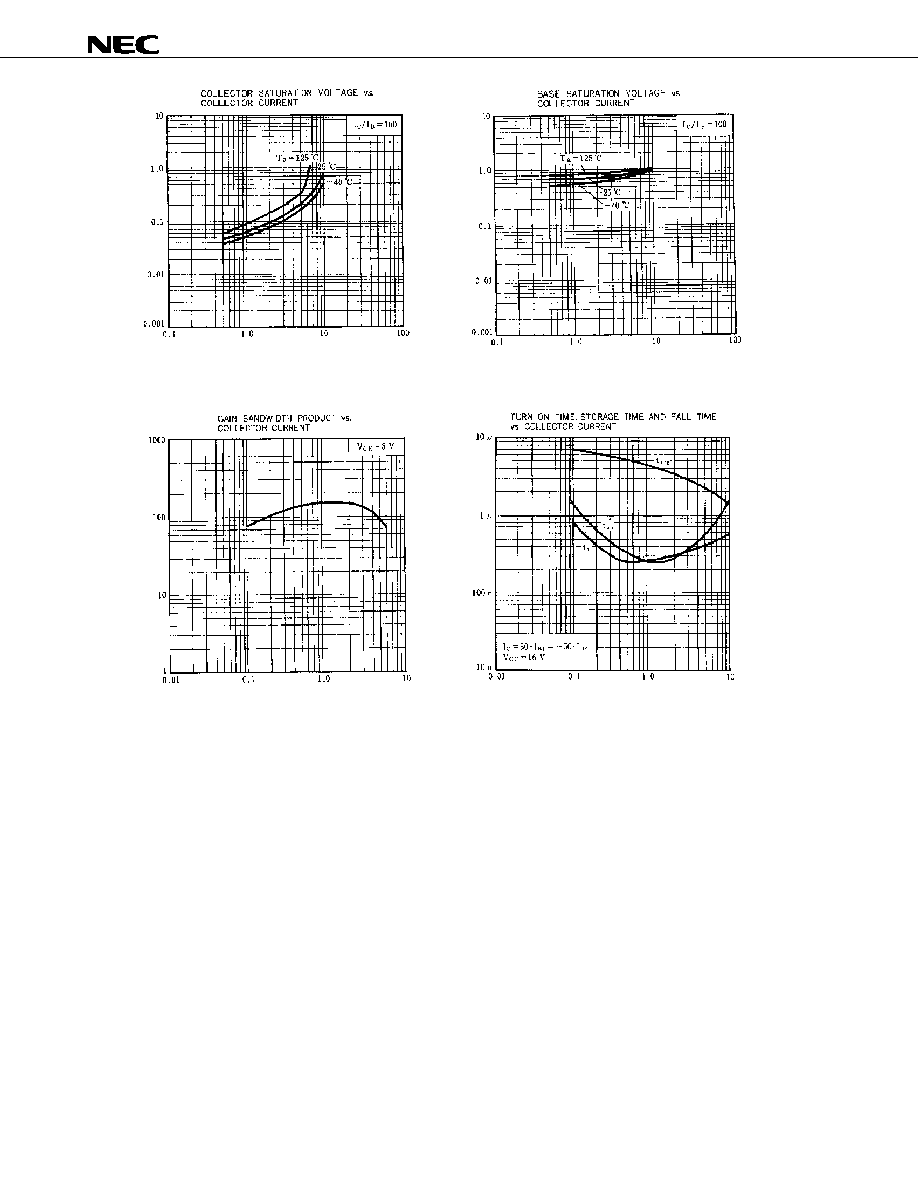Data Sheet D15603EJ2V0DS
4
2SC4813
Collector Current I
C
(A)
Collector Current I
C
(A)
Collector Current I
C
(A)
Col
l
e
c
t
or S
a
t
u
ra
t
i
on V
o
l
t
age
V
CE
(
s
a
t
)
(V
)
B
a
s
e

S
a
t
u
ra
t
i
on V
o
l
t
age V
BE
(s
a
t
)
(
V
)
Gai
n
B
andw
i
d
t
h
P
r
odu
c
t
f
T

(M
Hz)
Sw
itc
h
in
g
T
i
m
e
t
sw
(s
)
Collector Current I
C
(A)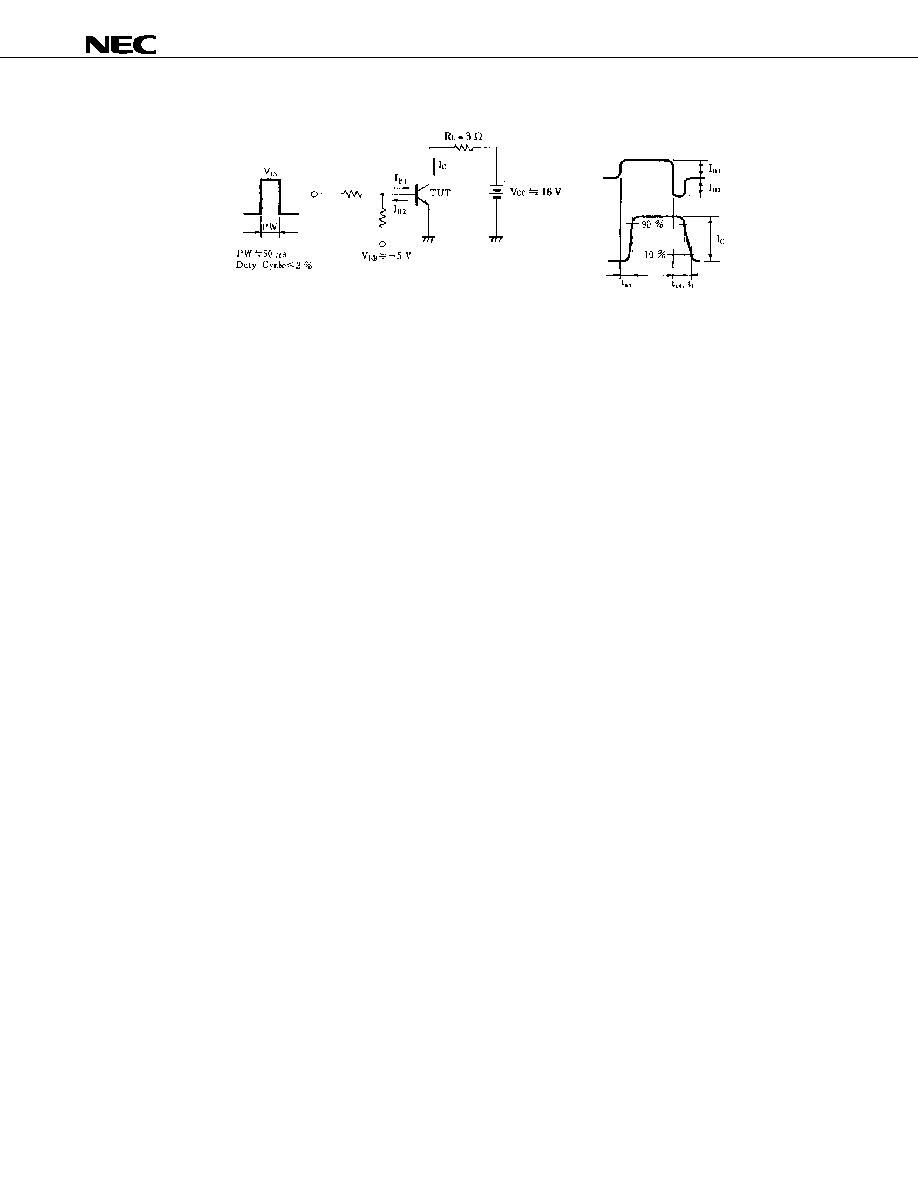Data Sheet D15603EJ2V0DS
5
2SC4813
SWITCHING TIME (t
on
, t
stg
, t
f
) TEST CIRCUIT
Base current
waveform
Collector current
waveform© 2019 • ChipFind• Контакты• Главная страница